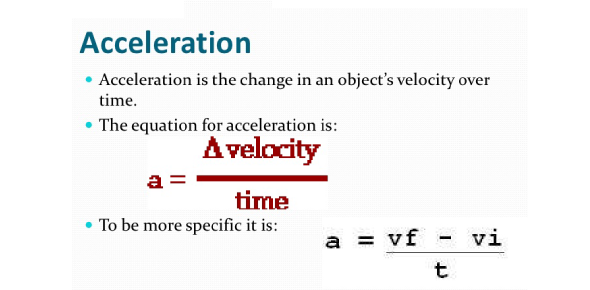# Trivia On Velocity And Acceleration! Quiz Questions

10 Questions | Total Attempts: 351SettingsDo you know anything about velocity and acceleration? Velocity is the rate of change of position concerning time, whereas acceleration is the rate of change of velocity. In mechanics, acceleration is the rate of the change in velocity of an object over time, divided by a period's duration. If you would like to discover further about velocity and acceleration, this is the quiz for you.

• 1.
Fast Freddie drives his race car 150m in 14.5 sec.  What is his average speed?
• 2.
In the graph below, how far has the object gone between segment b and c?
• 3.
In Graph C, which walker is walking faster, red or blue?
• 4.
What is the average speed for the blue walker in graph B?
• 5.
Calculate the acceleration of a car that goes from 30 m/s to 65 m/s in 5 sec.
• 6.
A model rocket starts on a launch pad.  It ascends at a speed of 3000 ft/s in 5 sec.  What is it's acceleration?
• 7.
According to tests, a Dodge Sprirt R/T can accelerate at 9.2 mi/hr/s.  How much time does it take to go from 0 mi/hr to 60 mi/hr?
• 8.
A hockey player skating at 18 m/s comes to a complete stop in 3 sec. What is his acceleration?
• 9.
What is the average speed between 0 and 15 sec?
• 10.
Where is the walker moving faster; between b-c or c-d?
Related TopicsBack to top